# Selina Solutions Concise Maths Class 10 Chapter 7 Ratio and Proportion Exercise 7(B)

Proportion and continued proportion are the main topics covered in the exercise. Students having any trouble in understanding these concepts can take help from the Selina Solutions for Class 10 Maths prepared by the subject experts at BYJUS. For any clarifications on the solutions of this exercise, the Concise Selina Solutions for Class 10 Maths Chapter 7 Ratio and Proportion Exercise 7(B) PDF is available in the links provided below.

## Selina Solutions Concise Maths Class 10 Chapter 7 Ratio and Proportion Exercise 7(B) Download PDF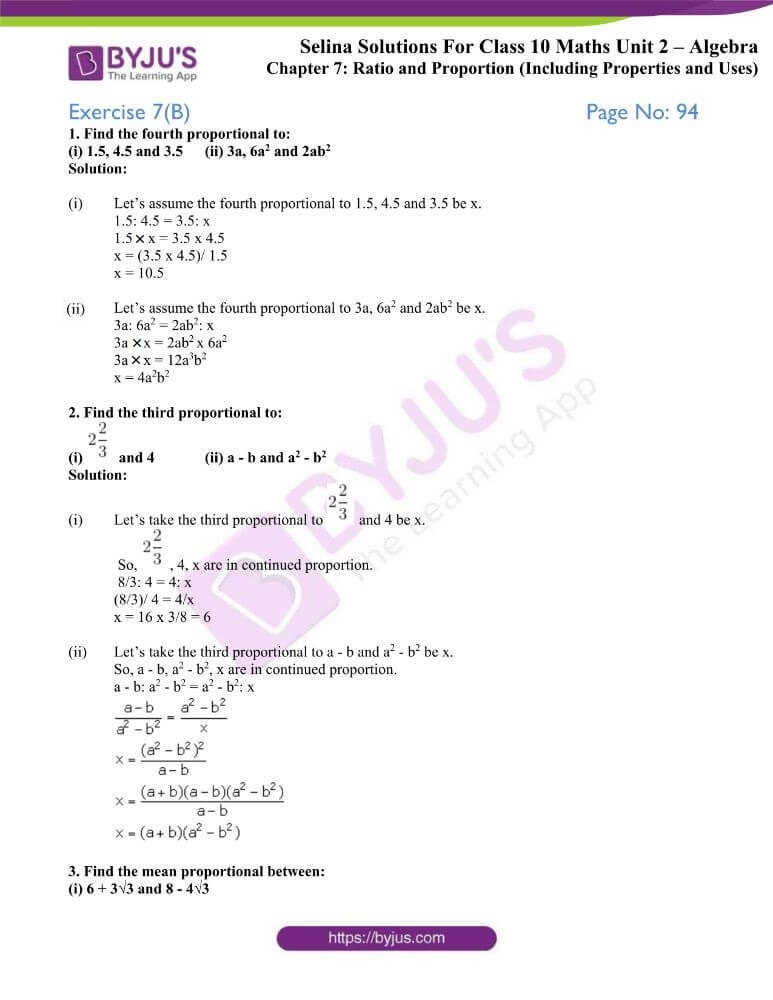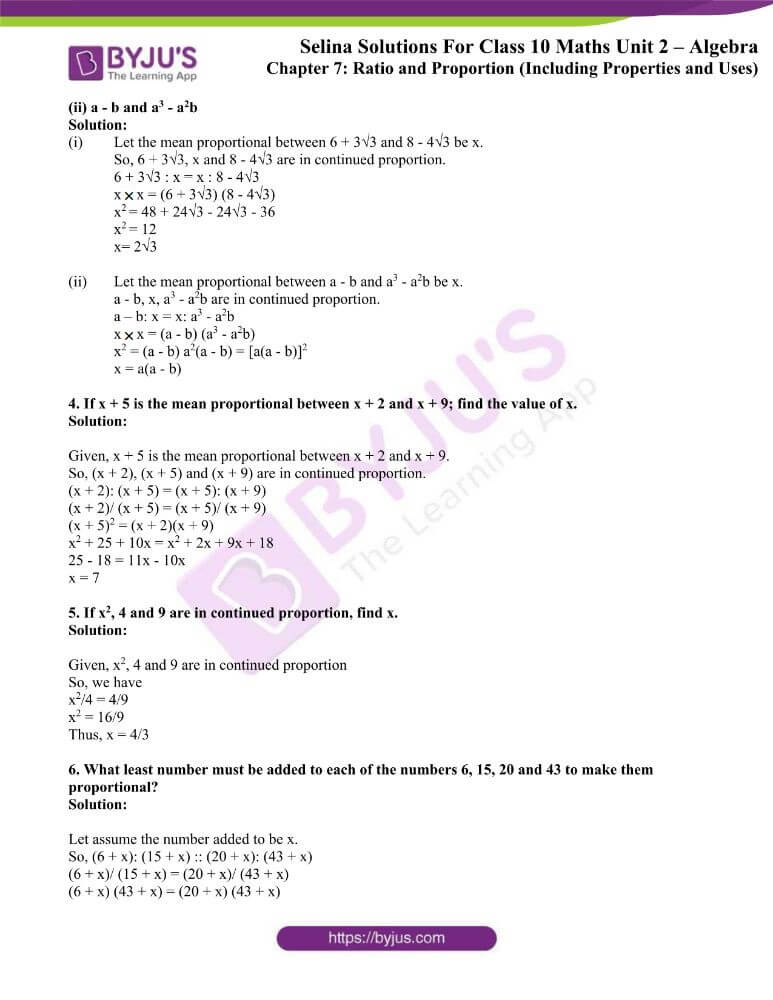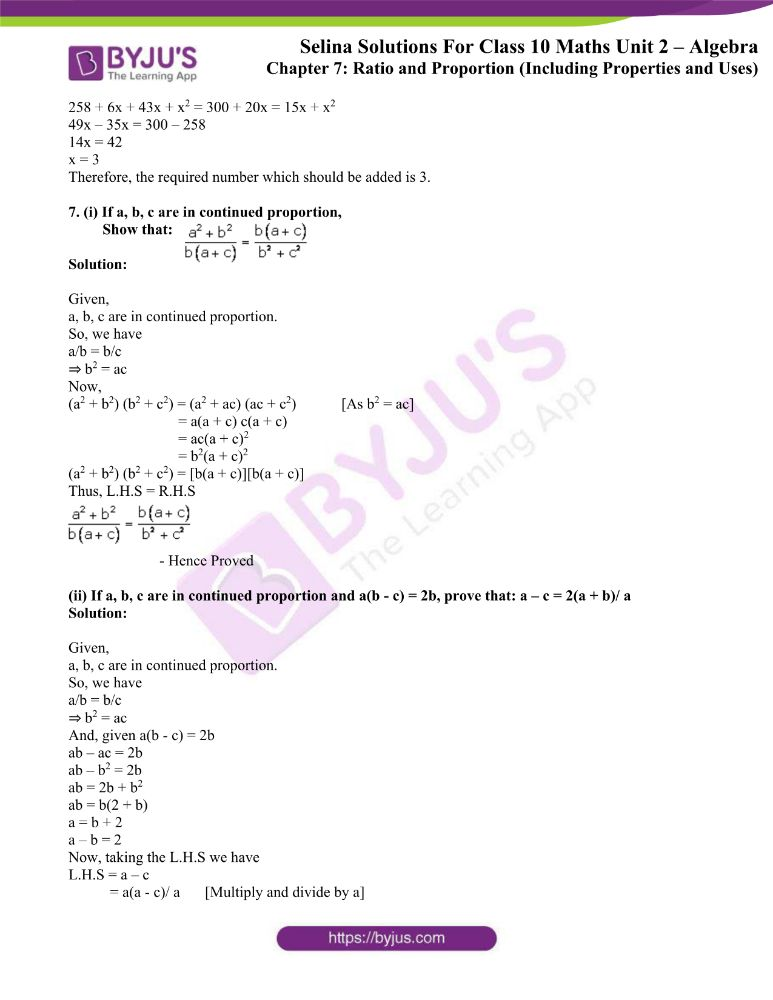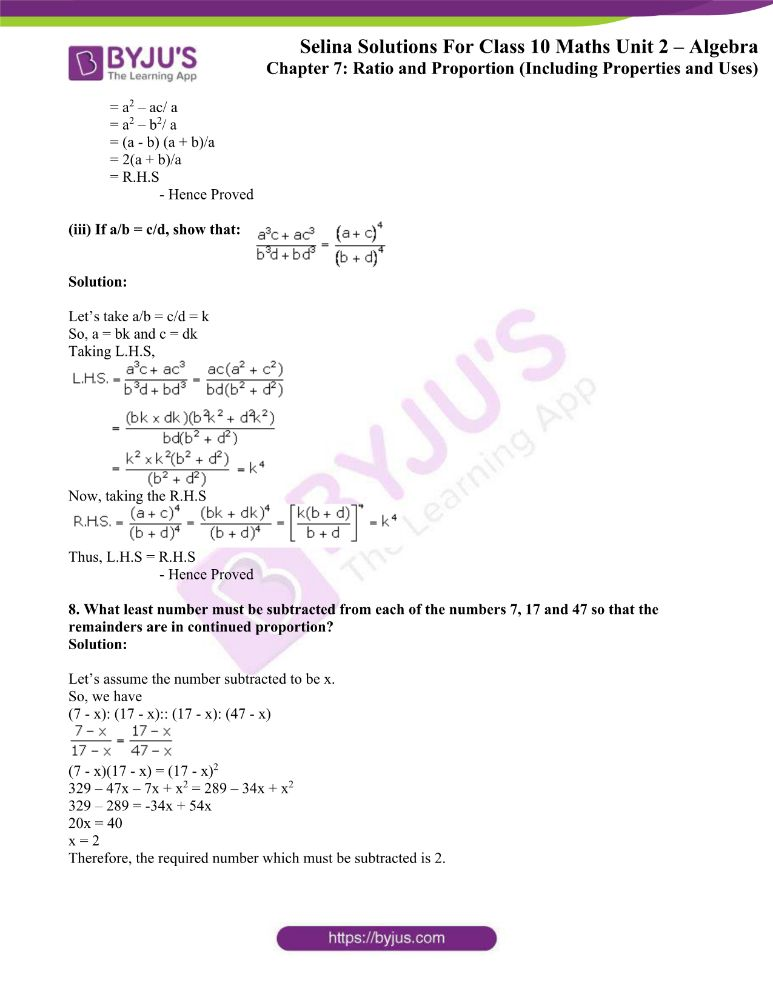### Access other exercises of Selina Solutions Concise Maths Class 10 Chapter 7 Ratio and Proportion

Exercise 7(A) Solutions

Exercise 7(C) Solutions

Exercise 7(D) Solutions

### Access Selina Solutions Concise Maths Class 10 Chapter 7 Ratio and Proportion Exercise 7(B)

1. Find the fourth proportional to:

(i) 1.5, 4.5 and 3.5 (ii) 3a, 6a2 and 2ab2

Solution:

(i) Let’s assume the fourth proportional to 1.5, 4.5 and 3.5 be x.

1.5: 4.5 = 3.5: x

1.5 × x = 3.5 x 4.5

x = (3.5 x 4.5)/ 1.5

x = 10.5

(ii) Let’s assume the fourth proportional to 3a, 6a2 and 2ab2 be x.

3a: 6a2 = 2ab2: x

3a × x = 2ab2 x 6a2

3a × x = 12a3b2

x = 4a2b2

2. Find the third proportional to:

(i)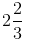and 4 (ii) a – b and a2 – b2

Solution:

(i) Let’s take the third proportional toand 4 be x.

So,, 4, x are in continued proportion.

8/3: 4 = 4: x

(8/3)/ 4 = 4/x

x = 16 x 3/8 = 6

(ii) Let’s take the third proportional to a – b and a2 – b2 be x.

So, a – b, a2 – b2, x are in continued proportion.

a – b: a2 – b2 = a2 – b2: x3. Find the mean proportional between:

(i) 6 + 3√3 and 8 – 4√3

(ii) a – b and a3 – a2b

Solution:

(i) Let the mean proportional between 6 + 3√3 and 8 – 4√3 be x.

So, 6 + 3√3, x and 8 – 4√3 are in continued proportion.

6 + 3√3 : x = x : 8 – 4√3

x × x = (6 + 3√3) (8 – 4√3)

x= 48 + 24√3 – 24√3 – 36

x= 12

x= 2√3

(ii) Let the mean proportional between a – b and a3 – a2b be x.

a – b, x, a3 – a2b are in continued proportion.

a – b: x = x: a3 – a2b

x × x = (a – b) (a3 – a2b)

x2 = (a – b) a2(a – b) = [a(a – b)]2

x = a(a – b)

4. If x + 5 is the mean proportional between x + 2 and x + 9; find the value of x.

Solution:

Given, x + 5 is the mean proportional between x + 2 and x + 9.

So, (x + 2), (x + 5) and (x + 9) are in continued proportion.

(x + 2): (x + 5) = (x + 5): (x + 9)

(x + 2)/ (x + 5) = (x + 5)/ (x + 9)

(x + 5)2 = (x + 2)(x + 9)

x2 + 25 + 10x = x2 + 2x + 9x + 18

25 – 18 = 11x – 10x

x = 7

5. If x2, 4 and 9 are in continued proportion, find x.

Solution:

Given, x2, 4 and 9 are in continued proportion

So, we have

x2/4 = 4/9

x2 = 16/9

Thus, x = 4/3

6. What least number must be added to each of the numbers 6, 15, 20 and 43 to make them proportional?

Solution:

Let assume the number added to be x.

So, (6 + x): (15 + x) :: (20 + x): (43 + x)

(6 + x)/ (15 + x) = (20 + x)/ (43 + x)

(6 + x) (43 + x) = (20 + x) (43 + x)

258 + 6x + 43x + x2 = 300 + 20x = 15x + x2

49x – 35x = 300 – 258

14x = 42

x = 3

Therefore, the required number which should be added is 3.

7. (i) If a, b, c are in continued proportion,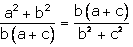Show that:

Solution:

Given,

a, b, c are in continued proportion.

So, we have

a/b = b/c

⇒ b2 = ac

Now,

(a2 + b2) (b2 + c2) = (a2 + ac) (ac + c2) [As b2 = ac]

= a(a + c) c(a + c)

= ac(a + c)2

= b2(a + c)2

(a2 + b2) (b2 + c2) = [b(a + c)][b(a + c)]

Thus, L.H.S = R.H.S– Hence Proved

(ii) If a, b, c are in continued proportion and a(b – c) = 2b, prove that: a – c = 2(a + b)/ a

Solution:

Given,

a, b, c are in continued proportion.

So, we have

a/b = b/c

⇒ b2 = ac

And, given a(b – c) = 2b

ab – ac = 2b

ab – b2 = 2b

ab = 2b + b2

ab = b(2 + b)

a = b + 2

a – b = 2

Now, taking the L.H.S we have

L.H.S = a – c

= a(a – c)/ a [Multiply and divide by a]

= a2 – ac/ a

= a2 – b2/ a

= (a – b) (a + b)/a

= 2(a + b)/a

= R.H.S

– Hence Proved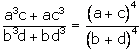(iii) If a/b = c/d, show that:

Solution:

Let’s take a/b = c/d = k

So, a = bk and c = dk

Taking L.H.S,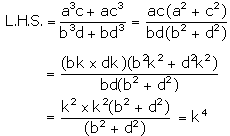Now, taking the R.H.S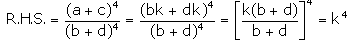Thus, L.H.S = R.H.S

– Hence Proved

8. What least number must be subtracted from each of the numbers 7, 17 and 47 so that the remainders are in continued proportion?

Solution:

Let’s assume the number subtracted to be x.

So, we have

(7 – x): (17 – x):: (17 – x): (47 – x)(7 – x)(17 – x) = (17 – x)2

329 – 47x – 7x + x2 = 289 – 34x + x2

329 – 289 = -34x + 54x

20x = 40

x = 2

Therefore, the required number which must be subtracted is 2.